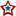#MN-Sen: Recount Regression, Day 2

For a more complete explanation of the process used, please refer to my initial post on the subject.

The following two graphs depict the number of votes gained or lost with respect to the total votes recorded for each precinct that has completed their recount. Each dot represents the change between the originally certified result and the post recount tally in a given precinct with x number of total votes:And the current functions used in conjunction with the regression:Now again using those functions, the following two graphs further illustrate the regression interpolation. The graph below illustrates the regression on precincts whose vote totals are less than 3,494; the largest precinct that has completed a recount. The dotted lines represent the previous day's regression.The graph below goes further and fits the regression onto all 4,130 precincts statewide; this forces 32,186 additional votes, that lie in precincts that surpass the 3,494 vote threshold, into the predetermined trend.Using the previous two graphs, and the functions they represent, a projection can be made for the cases covered. The first case simply includes precincts with 3,494 or less, while precincts above and beyond that figure are entirely ignored. For each precinct, the total vote total is taken and applied to the listed function for each candidate. The result is then added to that candidate's sum, and the next precinct is calculated. This process is done using precinct results from the final certification.

```            Coleman Gain: -514.680649876274  (+/- 8)
Franken Gain: -295.310532175405  (+/- 4)

Franken Net: 219.37011770087  (+/- 12)
```

Franken makes up the pre-recount deficit of 215 votes, while 32,186 votes are entirely ignored. If those additional votes are applied to the process, Franken adds an additional 9 votes:

```           Coleman Gain: -539.655665129103  (+/- 1648)
Franken Gain: -311.596023329002  (+/- 1916)

Franken Net: 228.059641800101  (+/- 3564)
```

As of right now (9:20 PM CT 11/20), after 42.33% of all votes have been recounted. Check back tomorrow for the day 3 result.

## 0 Response(s) to MN-Sen: Recount Regression, Day 2

### Leave a Reply:

Name: (Defaults to Anonymous)
Type the characters you see in the image below:(Word Verification)